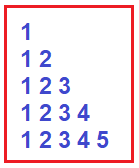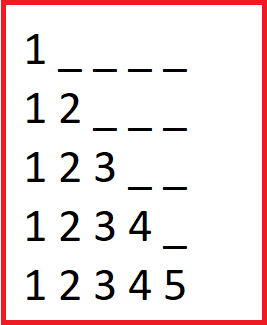# Half Pyramid of Numbers Program in C#

## Half Pyramid of Numbers Pattern Program in C#

In this article, I am going to discuss How to implement the Half Pyramid of Numbers Pattern Program in C# with Examples. Please read our previous article where we discussed the Inverted Pyramid of Numbers Pattern Program in C#. Please have a look at the below image which shows the Half-Inverted Pyramid of Numbers Pattern.##### Understanding Half Pyramid of Numbers Pattern in C#
1. In the first row, we want 1 and 4 blank spaces.
2. In the second row, we want 1 and 2, and 3 blank spaces.
3. In the third row, we want 1,2 and 3, and 2 blank spaces.
4. In the fourth row, we want 1,2,3, 4, and 1 blank space.
5. In the fifth row, we want 1,2,3,4, 5, and 0 blank spaces.
6. This list will go on as on the number of rows.1. From the above pattern, it is clear that at each row numbers are increasing +1 at each row.
2. For example, the 1st row has 1, the 2nd row has 1 and 2 and the 3rd row has 1,2, and 3, and so on.
3. From the above pattern, it is clear that in each row number of blank spaces is decreasing by –1 at each row. For example, the 1st row has 4 spaces, the 2nd row has 3 spaces, the 3rd row has 2 spaces, and so on.
##### How to implement the Half Pyramid of Numbers Pattern Program in C#?

To solve the above pattern,

1. We are going to use two for loops. The outer for loop and the inner for loop.
2. The outer for loop will be used to handle the rows one by one and the inner for loop will be used to handle the columns one by one.
3. From the above explanation, it is clear that the number in each row is equal to the current row.
4. 1st row has 1, 2nd row has 1 and 2, and so on.
5. The inner loop will be used to print the numbers according to row and the outer loop’s job is to go on a new line after printing the current row numbers.
##### Program to Print Half Pyramid of Numbers Pattern in C#

The following C# Program will allow the user to input the number of rows and then print the Half Pyramid of Numbers Pattern on the console.

```using System;
namespace PatternDemo
{
public class HalfPyramidOfNumbersPattern
{
public static void Main()
{
Console.Write("Enter number of rows :");
for (int i = 1; i <= rows; i++)
{
for (int j = 1; j <= i; j++)
{
Console.Write(j + " ");
}
Console.WriteLine("\n");
}